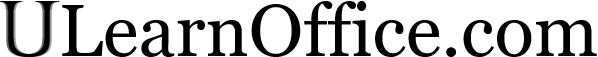Previous
Next
Homepage
FAQ
What's new
Sitemap

TopicsIntroduction
Basic commands
Presentation
Page layout and printing
Functions
Copy, Paste and
relative and absolute references

Charts (graphics)
Macro commands
Analysis tools
Data list
Pivot table
Exercise: creating a model
Quiz

TutorialsWord
Excel

Excel 2007/2010/2013
PowerPoint
Access

OthersDemonstration files
Texte en français

ContactBy e-mail

# Excel - Copy, Paste and references

## Introduction

To effectively use a spreadsheet, you must be able to create formulas to calculate automatically any change that you could bring to your model. Because some of these formulas are alike, it's very effectient to copy them than to rewrite them. This page demonstrates how to copy formulas and also looks at the very important point of relative and absolute references. You must master these notions to properly use a spreadsheet.

## Copy

One of the big advantages of a spreadsheet is to be able to copy a formula or a text as often as you need to create a model. It's easier and faster to copy a formula than to rewrite it every time you need it. The usual way to copy a cell or a range of cells is as follows.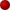Select the cell or the range of cells to be copied.From the Edit menu, select the Copy option.
ORPress thebutton.
ORPress the CTRL and C keys.Move the cursor in the cell you want to copy the content or make a range of cells where you want to copy the formula.From the Edit menu, select the Paste option.
ORPress the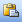button.
ORPress the CTRL and V keys.

Another technique to copy is to use the Autofill handle located in the lower right corner of the cell or a range of selected cells.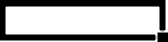You need to:Place the cursor in the cell to be copied.Place the cursor on the small black square in the lower right corner of the cell.Press the left mouse button and move the cursor to the right.

The contents of the cell will be copied into the selected cells. You can also copy the contents in any direction: left, right, up or down. You can also copy a range of cells at the same time. The result will vary as the contents of cells are numbers or formulas. There is an exercise on this option later on this page.

## Absolute and relative reference: IMPORTANT

Coping formulas is the fastest way to create a model. There is however a trap that you must be aware. Let's place the following formula =A1+A2 in the A3 cell. If you copy this formula in the B3 and C3 cells, the result would be the following: B3: =B1+B2 , C3: =C1+C2. Why did Excel not copy exactly the formula =A1+A2?

Actually, it did copy the formula. It's all in the way that Excel interprets the formula. It's not the cell's address that's important but its position with regards to the cells where the formula is located. If the formula is in the A3 cell, Excel will interpret the formula =A1+A2 this way: the A1 cell is two cells above the current position of the formula and A2 cell is just above. So, when Excel will copy the formula it's going to add both cells just above where the formula is placed. It's for that reason that the formulas copied in the B3 (=B1+B2) and C3 (=C1+C2) cells are this way. They also add the numbers of the two cells above the formula. This kind of reference in the formula is called relative reference. Everything is relative to the location of the formula. Excel always fetches the numbers in the same way. It's also more practical when you copy a formula. You usually use the same formula on a different set of numbers. For example, you can copy a formula that adds the monthly expenses. The same formula that was needed for January should also work for every other month of the year.

Quiz: If you copy the formula =A1+A2 from the A3 cell to the Z20 cell, what will the formula look like? The answer is at the end of this page.

But what can you do when you need a variable in the formula? A variable is information that can change at any time. For example, if you want to know what happens to the profits if the growth rate changes from 5 % to 50 %. Or what if the number of employees passes from 5 to 25? Or what if the mortgage rate to be renewed changes?

A model consists of three elements: constants, variables and formulas. Constants are number needed to start your model. They will not change during the timeline of your model. Even these numbers change in the long run, but not in the time frame for this model. Examples of constants are tax and insurance rates, sales tax, telephone bill and mortgage payments.

Variables are the data that you want to test the model by asking " what happens if... " questions. What will happen if the interest rates rise? What will happen if a customer doesn't pay? What will happen at such a growth rate? These variables can change at any time so that you can try to find the best solutions for your model.

Never write a variable in a formula. For example, never write =100000*10%. Otherwise, you'll have to change every formula each time the variable changes. It's for that reason that you need to place a variable in a single cell. If the variable is written in several cells, chances are you will forget to change a value in one of these cells. This would invalidate your entire model because you forgot to change a single value. It also makes it very difficult to make sure that the model is correct because you must verify all the cells or where the variable is located. This would make the validation process, very long and very painful. So, it's better to avoid copying a variable in several places in a file. The best way is to write a variable in a single cell and have every formula refer to that cell. Change the content of a single cell and your entire model changes! Isn't it why you created a model in the first place?

This makes the work of creating formulas a little more complicated. What must you do when you want to copy a formula? Cell addresses are composed of two components: a reference to the column and to row they're located. The first cell's address, A1, is composed of these two components. The letter represents the column and the number represents the row. The column's addresses are from A to Z and then from AA to IV (in Excel 2007 it goes up to XFD). The row's addresses are from 1 to 65 565 (compared to Excel 2007's 1 048 576).

By copying a formula, the relative reference changes too. The column reference changes when you copy a formula horizontally (left to right). The row reference changes when you copy a formula verticaly (up and down).

You can use the dollar sign (\$) to "fix" or "freeze" a column or a row in a formula. When the formula will be copied, the parts that are frozen will not change. To freeze, you must place the dollar sign (\$) in front of the column or row position.

 A1 Neither the column nor the row is frozen. \$A1 The column is frozen but not the row. A\$1 The row is frozen but not the column. \$A\$1 The row and the column are frozen.

Example:

Write numbers and following formula in the appropriate cells.
A1: 100 , A2: 200 , A3: =\$A1+A2

Copy the contents of the A3 cell in the B3 to E3 cells. There are many ways to copy the formula. Here are two of themPlace the cursor in the A3 cell.From the Edit menu, select the Copy option.Make a range with the B3 to E3 cells.From the Edit menu, select the Paste option

There is also the other way by using the fill handle.Place the cursor in the A3 cell.Place the cursor on the lower right corner of the cell, on the black square in the corner. The shape of the cursor should change to a black "+" sign.Keep your finger on the left mouse button and move the cursor up to the E3 cell.

The other ways would have been to use the CTRL+C and CTRL+V keys to copy and paste the cell. You could also have used the copy and paste buttons on the standard toolbar or use the copy and paste options from the context menu that's available by pressing the right mouse button.

The formulas in the B3 to E3 cells are:

 Cell Result Formula B3 100 =\$A1+B2 C3 100 =\$A1+C2 D3 100 =\$A1+D2 E3 100 =\$A1+E2

By "freezing" the A column in the formula, this reference will always look for the value in the first column (A). This is necessary when you want to look for the contents of a cell that contains a variable and you wish to copy the formula. A formula that has a relative position would not allow you to look for the contents of the A1 cell once it's copied elsewhere. By freezing the column or the row in the formula, you'll not be obliged to change the formula once you copied it to other cells.

If you want to copy a formula horizontally, you may only need to freeze the column reference (\$A1). If you wish to copy a formula vertically, you may need to freeze the row or row reference (A\$1). If you a coping the formula to a different row and column, you may need to freeze both. It depends on where the data is located relative to the position of your formula. If they're always in the same cell, you need to freeze the column of row to get an absolute reference. Excel will always look for the data in the same column or row.

## Exercise with the exchange rate

This exercise is to demonstrate the enormous advantage of using relative and absolute references in formulas that you want to copy. It consists in changing the values of US currency into Canadian dollars.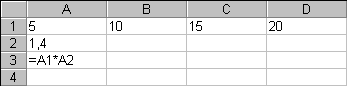Write numbers and formulas in the appropriate cells.

For this exercise, we presume that 1 US dollar equals 1.4 Canadian dollars, which is a the rate in the A2 cell. The A3 cell contains the formula that will help us to find the value for the first column. The result of this cell should be 7 (5 * 1.4 = 7). You could write the formula again for the following cells, from B3 to D3, but it's even more effective to copy the formula to the other cells (B3 to D3).Place the cursor in the A3 cell.From the Edit menu, select the Copy option.
ORPress thebutton.
ORPress the CTRL and C keys.

The frame around the A3 cell will flash. This is normal. It's to remind you that it's the cell that you have just copied.Make a range with the B3 to D3 cells.From the Edit menu, select the Paste option.
ORPress thebutton.
ORPress the CTRL and V keys.

And here it's! The formula is now copied in the other cells that you have just specified. However, the result is not what you expected. There are only zeros instead of values! Let's find out why.Place the cursor in the B3 cell.

You can see from the formula bar the following contents: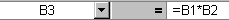You'll notice that the B1 cell contains a value ( 10 ) whereas the B2 cell is empty. It's for that reason that Excel shows the value 0 (10 * 0 = 0) in the B3 cell.

To solve this small problem, you could copy the exchange rate of the A2 cell in the B2 to D2 cells. But that's not a practical solution for several reasons. In the first place, the same value would occupy several cells for nothing. You could put the other numbers or formulas in that place. Secondly, the chances to forget to change the contents of a single cell during modifications are far too high. It would be really pity to have a useless model because you forgot to change the contents of one or two cells!

It's preferable to put the values of variables and constants in cells apart and adjust formulas. It's never a good idea to put the value of a constant or a variable in a formula. As mentioned in the previous paragraph, if you forget to change the value in a single cell, invalidates your model.

In fact constants and variables have something common: they can change. A variable can change any time for the needs of your model: occupation rate, price of a product, price of the material etc. A constant can also change but on a mid to long-term basis: taxes, tax rates, interest rates... Because a variable or constant can change, it's much easier to manage it by placing the value in a single cell. You can adjust your formulas to refer to that cell using relative and absolute references. You must master theses references if you wish to copy formulas and efficiently use Excel or any other spreadsheet.

Let's return to the exercise. The formula must be adjusted so that it can always find the exchange rate in the right cell; A2 in our box. But what must you know before adjusting the formula?

Is there a part of the formula that you want to fix or freeze? For the exercise, you must "fix" the A2 part of the formula.

You must know if you will copy the formula horizontally, vertically or both? For the exercise, the formula will be copied horizontally in the B3 to D3 cells. Because it's horizontally, you only need "freeze" or to "fix" the letter of the column and not the number of the row in the cell address contained in the formula.

It's now time to change the formula of the A3 cell to adapt it.Place the cursor in the A3 cell.

To change the contents of the cell.Press the F2 key.
ORDouble-click the A3 cell.
ORPlace then the cursor on the formula bar.Change the contents of the formula to this: =A1*\$A2

The formula was changed but the value remains the same: 7 (5 * 1,4). It's only when you'll copy the formula and see the results that you'll notice the change.Copy the formula in the A3 cell into the B3 to D3 cells.

If you need a reminder, refer to the instructions for copying above.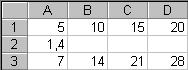Verify the formulas of in the B3 to D3 cells.

B3: =B1*\$A2
C3: =C1*\$A2
D3: =D1*\$A2

You have now the right result because you "froze" the A column of the A2 cell. Look how it practical it can be:Change the value of the A2 cell from 1.4 to 1.25.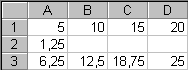All the values in worksheet have been changed by changing the content of a single cell! What do you prefer now; change the contents of a single cell or change several cells? Which one is the most efficient? (Answer: change a single cell)

But you must pay attention in the way that you use the relative and absolute references.Place the cursor in the A3 cell.Copy the contents of the cell.Paste it in the A4 to A8 cells.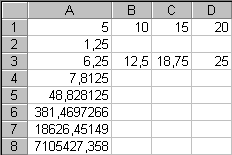What exchange rate! Let's verify the contents of the A4 to A8 cells.

A4: = A2*\$A3
A5: =A3*\$A4
A6: =A4*\$A5
A7: =A5*\$A6
A8: =A6*\$A7

You see the problem! The formula was copied vertically and not horizontally. Although the column is frozen, the rows are not. It's for that reason that the results climb so quickly. Be careful when you apply relative and absolute references. Be sure to freeze the right column or row.

### Video: Excercice on cell rferencing with Vlookup() function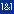This site is hosted by 1&1.com